# Mechanical Engineering - Heat Transfer, Refrigeration and Air Conditioning

### Exercise :: Heat Transfer, Refrigeration and Air Conditioning - Section 7

1.

Sensible heat factor is given by (where S.H. = Sensible heat, and L.H. = Latent heat)

 A.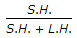B.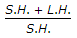C.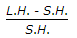D.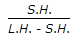Explanation:

No answer description available for this question. Let us discuss.

2.

Stefan-Boltzmann law is applicable for heat transfer by convection.

 A. Agree B. Disagree

Explanation:

No answer description available for this question. Let us discuss.

3.

The most commonly used method for the design of duct size is the

 A. velocity reduction method B. equal friction method C. static regain method D. dual or double method

Explanation:

No answer description available for this question. Let us discuss.

4.

In counter-current flow heat exchangers

 A. both the fluids at inlet are in their hottest state B. both the fluids at inlet are in their coldest state C. both the fluids at exit are in their hottest state D. one fluid is coldest and the other is hottest at inlet

Explanation:

No answer description available for this question. Let us discuss.

5.

The rate of heat flow through a body is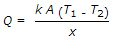. The term x/kA is known as

 A. thermal coefficient B. thermal resistance C. thermal conductivity D. none of these